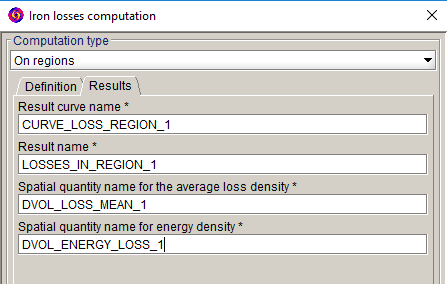# Results of iron losses computation on regions

## Computation results

The computation of iron losses on regions gives the following results## Curve

"CURVE_LOSS_REGION_i" curve can be displayed automatically and is stored in the tree in the area Postprocessing > Curve > 2D curve (I/O Parameter).

It represents instantaneous losses on the region(s) versus time.

## Result

The results are automatically displayed in the data tree in the area Postprocessing > Result > Iron losses Result.

The results include:
• Average losses over a period on region(s) (in W)
• Iron losses energy on region(s) (in J)
• Error on periodicity (in %)

## Spatial quantities

Spatial quantities, denominated "DVOL_ENERGY_LOSS_i" and "DVOL_LOSS_MEAN_ i" are stored in the data tree in the area Parameter/Quantity > Spatial quantity

Spatial quantities correspond to:
• Average loss density “DVOL_LOSS_MEAN_i”
• Losses energy density "DVOL_ENERGY_LOSS_i"

## Display loss density (isovalues)

It is possible to display the above mentioned spatial quantities in a color shade graphic (isovalues).

For this: